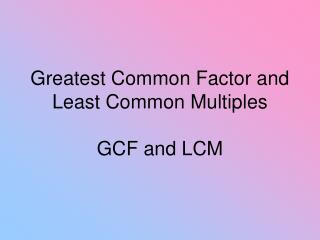DownloadDownload PresentationGreatest Common Factor and Least Common Multiples GCF and LCM

# Greatest Common Factor and Least Common Multiples GCF and LCM

Download Presentation## Greatest Common Factor and Least Common Multiples GCF and LCM

- - - - - - - - - - - - - - - - - - - - - - - - - - - E N D - - - - - - - - - - - - - - - - - - - - - - - - - - -
##### Presentation Transcript

1. Greatest Common Factor and Least Common MultiplesGCF and LCM

2. What is the difference between a factor and a multiple?

3. Factor:An integer that divides evenly (no remainder) into a numberFactors of 6 are: 1, 2, 3, 6

4. Multiple:The product of a number and any natural numberSome multiples of 6: 6, 12, 18, 24……

5. Give me an example of a factor of 15

6. Give me an example of a multiple of 15

7. How would you find the GCF of 60 and 96?

8. You simply write out all the factors for each number. Then find the greatest number. I like to circle the numbers by using columns.

9. List the factors of 60:1,2,3,4,5,6,10,12,15,20,30,60List the factors of 961,2,3,4,6,8,12,16,24,32,48,96find the largest factor - 12

10. Find the GCF of 36 and 24

11. Find the LCM of 12 and 18

12. Multiples of 12 are…12,24,36,48,60,72,….Multiples of 18 are…18,36,54,72,90,108,…The smallest multiple the 2 numbers have in common is the least common multiple.

13. Find the LCM of 18 and 12